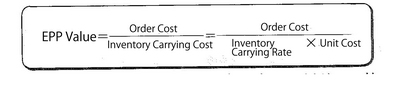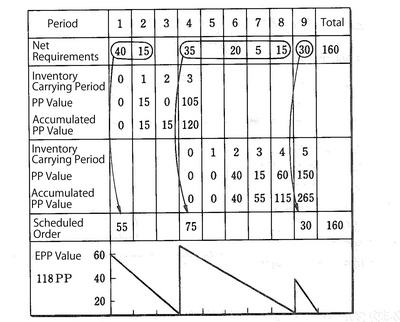#### Asprova MRP

Asprova MRP is calculated on the memory requirement, allocate lead time at a high speed. Can achieve material arrangement Just in Time, which is impossible in other MRP system.

MRP glossary TOP > Material Planning > PPB Method

## PPB Method

Part Period Balancing

Like EOQ, it is a method for lot sizing performed in order to implement the lowest cost in relationship between order cost and inventory carrying cost. Since lot sizing is performed by fluctuating the order quantity and the period number of sizing, however, it is also effective for the items whose demand is unstable while EOQ is suitable for the items whose demand is stable. In this method, a basic unit of PP (Part-Period) is used. When one part is stored for one period, the value is 1 PP, and the total value of all PP values calculated for each period is an accumulated PP. When accumulated inventory carrying cost excesses the order cost, the quantity of schedule order is set. In order to make these calculations easier, a standard value for lot sizing, called EPP (Economic Part-Period), is used. This value, meaning that the accumulated PP is over the order cost, is calculated by means of the following formula:In the Part Period Balancing, as the figure shows, lots are sized with up to one period before the time when the accumulated PP is over EPP.Related term: Lot Sizing

Reference：JIT Business Research Mr. Hirano Hiroyuki